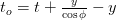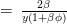# 超光速激光点

A discussion in the Science Forums on the appearance of a laser dot on a ceiling. It is thought that if you pointed a laser dot on a ceiling and turned the laser gun fast enough, you could create superluminal laser dots. Could you, 真?If a superluminal object did exist, 它会出现两个相同的对象给我们?$x = y\tan\phi = \beta t$$t = \frac{y\tan\phi}{\beta}$$t_o = t + \frac{y}{\cos\phi} - y$$t_o = y\left( \frac{\tan\phi}\beta + \frac{1}{\cos\phi} - 1\right)$$t_o = y\left(\frac\phi\beta + \frac{\phi^2}{2}\right)$
(调用这个公式Q.)$1+\frac{2\beta^2}{y}t_o = \left(1+\beta\phi\right)^2$

The angular separation between the objects flying away from each other is the difference between the roots of the quadratic equation Q:$\Phi \,=\, \phi_1-\phi_2$$\,=\, \frac{2}{\beta}\sqrt{1+\frac{2\beta^2}{y}t_o}$$\,=\, \frac{2}{\beta}\left(1+\beta\phi\right)$$\frac{d\Phi}{dt_o} \,=\, \frac{2\beta}{y\sqrt{1+\frac{2\beta^2}{y}t_o}}$$\,=\, \frac{2\beta}{y\left(1+\beta\phi\right)}$$\frac{d\Phi}{dt_o} \,=\, \frac{2beta}{y\sqrt{1+\frac{2\beta^2}{y}t_o}}$$\,=\, \frac{2\beta}{y\sqrt{1-\frac{t_o}{{t_o}_{min}}}}$$\,=\, \sqrt{\frac{4\beta^2}{y^2}\,\times\,\frac{-{t_o}_{min}}{t_o-{t_o}_{min}}}$$\,=\,\sqrt{\frac{2}{y, t_{age}}}$

• 其原因在右侧的点并不比C移动速度更快有密切关系方式所来源的相对论速度极限.
• 人们也应该考虑到，说上的点位于在在一定的时间瞬间的特定点天花板不够好. 当将观测 (想必在激光枪) 看看吧? 有轻旅行多了一个腿 (从点回枪) 需要包括. 这种考虑可能是同时性的定义背后 (利用光的往返行程) 在斯洛伐克.
• 到分离点的左侧 (黑点), 时间的流动是相反的. 换句话说, 如果你改变了彩色激光作为你的身影到中心左边缘扫描, 在该点的颜色的变化会出现在相反的顺序 (及时).
• 多普勒频移也被在该区域反转. 这就是为什么我很感兴趣的左手材料, 其中的群速度和多普勒频移被颠倒.